StatLect

# Score vector

In the theory of maximum likelihood estimation, the score vector (or simply, the score) is the gradient (i.e., the vector of first derivatives) of the log-likelihood function with respect to the parameters being estimated.## Definition

The concept is defined as follows.

Definition Letbe aparameter vector describing the distribution of a sample. Letbe the likelihood function of the sample, depending on the parameter. Letbe the log-likelihood function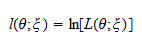Then, thevector of first derivatives ofwith respect to the entries of, denoted by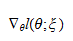is called the score vector.

The symbolis read nabla and is often used to denote the gradient of a function.

## Example

In the next example, the likelihood depends on aparameter. As a consequence, the score is avector.

Example Suppose the sampleis a vector ofdraws, ...,from a normal distribution with meanand variance. As proved in the lecture on maximum likelihood estimation of the parameters of a normal distribution, the log-likelihood of the sample is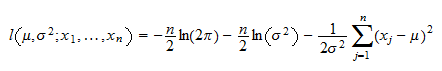The two parameters (mean and variance) together form avectorThe partial derivative of the log-likelihood with respect tois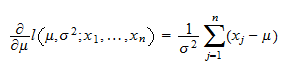and the partial derivative with respect to the varianceis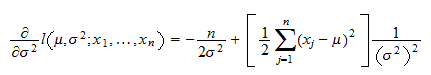The score vector is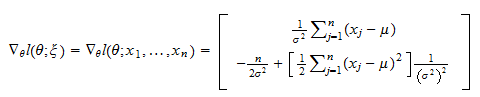## How the score is used to find the maximum likelihood estimator

The maximum likelihood estimatorof the parametersolves the maximization problem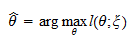Under some regularity conditions, the solution of this problem can be found by solving the first order conditionthat is, by equating the score vector to.

## More details

More details about the log-likelihood and the score vector can be found in the lecture entitled Maximum likelihood.